# Circle - JEE Main Previous Year Question with Solutions

JEE Main Previous Year Question of Math with Solutions are available at eSaral. Practicing JEE Main Previous Year Papers Questions of mathematics dwill help the JEE aspirants in realizing the question pattern as well as help in analyzing weak & strong areas. Get detailed Class 11th &12th Physics Notes to prepare for Boards as well as competitive exams like IIT JEE, NEET etc. eSaral helps the students in clearing and understanding each topic in a better way. eSaral is providing complete chapter-wise notes of Class 11th and 12th both for all subjects. Besides this, eSaral also offers NCERT Solutions, Previous year questions for JEE Main and Advance, Practice questions, Test Series for JEE Main, JEE Advanced and NEET, Important questions of Physics, Chemistry, Math, and Biology and many more. Download eSaral app for free study material and video tutorials.
Q. Three distinct points A, B and C are given in the 2–dimensional coordinate plane such that the ratio of the distance of any one of them from the point (1, 0) to the distance from the point (–1, 0) is equal to $\frac{1}{3}$. Then the circumcentre of the triangle ABC is at the point :- (1) $\left(\frac{5}{2}, 0\right)$ ( 2)$\left(\frac{5}{3}, 0\right)$ (3) (0, 0) ( 4)$\left(\frac{5}{4}, 0\right)$ [AIEEE-2009]
Ans. (4) Let $A, B, C$ are represented by the point $(x, y)$ $\frac{\sqrt{(x-1)^{2}+y^{2}}}{\sqrt{(x+1)^{2}+y^{2}}}=\frac{1}{2}$ $8 x^{2}+8 y^{2}-20 x+8=0$ Which is the circle which passes through the points $A, B, C$ then circumcentre will be the centre of the circle $\left(\frac{5}{4}, 0\right)$
Q. If $P$ and $Q$ are the points of intersection of the circles $x^{2}+y^{2}+3 x+7 y+2 p-5=0$ and $x^{2}+y^{2}+2 x+2 y-p^{2}=0,$ then there is a circle passing through $P, Q$ and $(1,1)$ is possible for : $-$ (1) All except two values of p (2) Exactly one value of p (3) All values of p (4) All except one value of p [AIEEE-2009]
Ans. (4) Equation of line $\mathrm{PQ}$ $\mathrm{x}+5 \mathrm{y}+2 \mathrm{p}-5+\mathrm{p}^{2}=0$ $\mathrm{P}, \mathrm{Q}$ and $(1,1)$ will not lie on a circle of $(1,1)$ Lies on the line $\mathrm{x}+5 \mathrm{y}+\mathrm{p}^{2}+2 \mathrm{p}-5=0$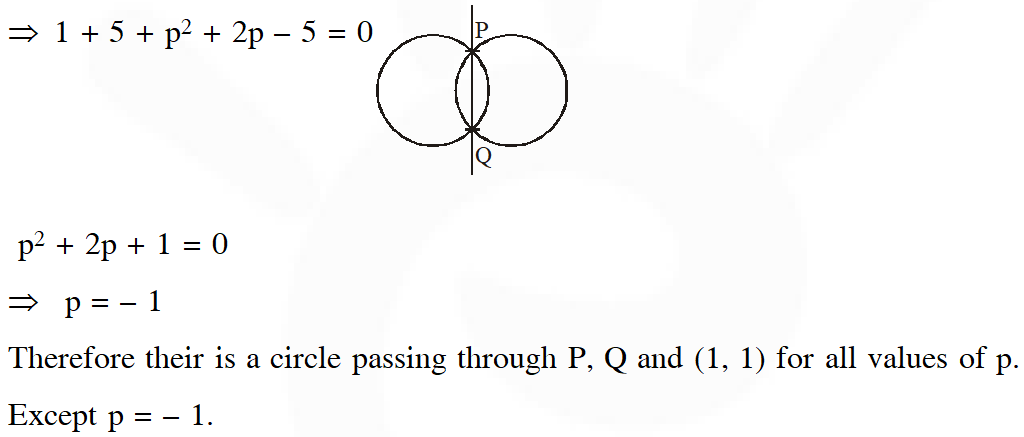Q. For a regular polygon, let r and R be the radii of the inscribed and the circumscribed circles. A false statement among the following is :- (1) There is a regular polygon with $\frac{\mathrm{r}}{\mathrm{R}}=\frac{1}{2}$ (2) There is a regular polygon with $\frac{r}{R}=\frac{1}{\sqrt{2}}$ (3) There is a regular polygon with $\frac{\mathrm{r}}{\mathrm{R}}=\frac{2}{3}$b (4) There is a regular polygon with $\frac{\mathrm{r}}{\mathrm{R}}=\frac{\sqrt{3}}{2}$ [AIEEE-2010]
Ans. (3)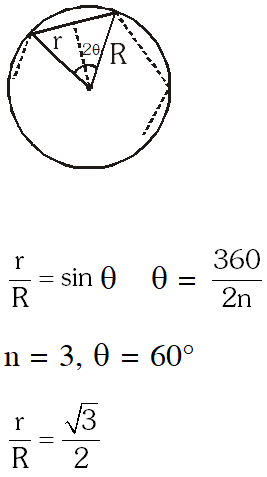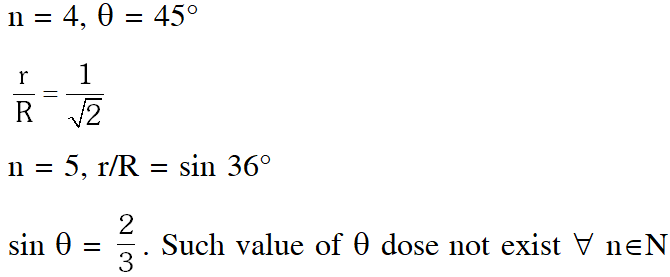Q. The circle $\mathrm{x}^{2}+\mathrm{y}^{2}=4 \mathrm{x}+8 \mathrm{y}+5$ intersects the line $3 \mathrm{x}-4 \mathrm{y}=\mathrm{m}$ at two distinct points if :- (1) – 85 < m < – 35 (2) – 35 < m < 15 (3) 15 < m < 65 (4) 35 < m < 85 [AIEEE-2010]
Ans. (2)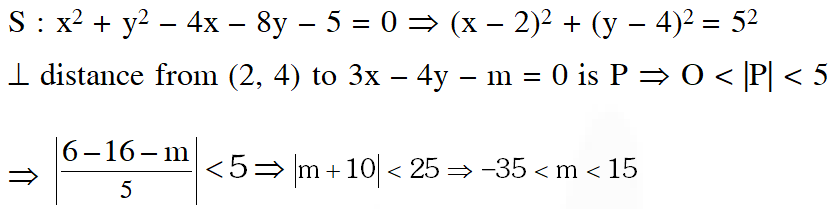Q. The two circles $\mathrm{x}^{2}+\mathrm{y}^{2}=\mathrm{ax}$ and $\mathrm{x}^{2}+\mathrm{y}^{2}=\mathrm{c}^{2}(\mathrm{c}>0)$ touch each other if :- (1) $\mathrm{a}=2 \mathrm{c}$ (2) $|\mathrm{a}|=2 \mathrm{c}$ (3) $2|\mathrm{a}|=\mathrm{c}$ (4) $|\mathrm{a}|=\mathrm{c}$ [AIEEE-2011]
Ans. (4)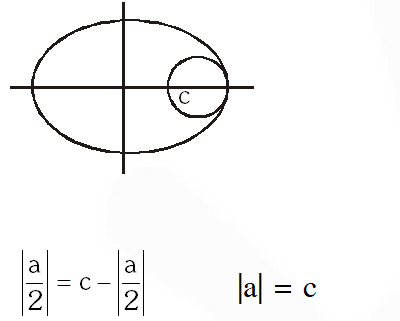Q. The equation of the circle passing through the points (1, 0) and (0, 1) and having the smallest radius is – (1) $x^{2}+y^{2}+x+y-2=0$ (2) $x^{2}+y^{2}-2 x-2 y+1=0$ (3) $x^{2}+y^{2}-x-y=0$ (4) $x^{2}+y^{2}+2 x+2 y-7=0$ [AIEEE-2011]
Ans. (3) (1, 0) and (0, 1) will be ends of diameter So equation of circle (x- 1) ( $\mathrm{x}-0)+(\mathrm{y}-0)(\mathrm{y}-1)$ $\mathrm{x}^{2}+\mathrm{y}^{2}-\mathrm{x}-\mathrm{y}=0$
Q. The length of the diameter of the circle which touches the x-axis at the point (1, 0) and passes through the point (2, 3) is : (1) 5/3           (2) 10/3            (3) 3/5             (4) 6/5 [AIEEE-2012]
Ans. (2)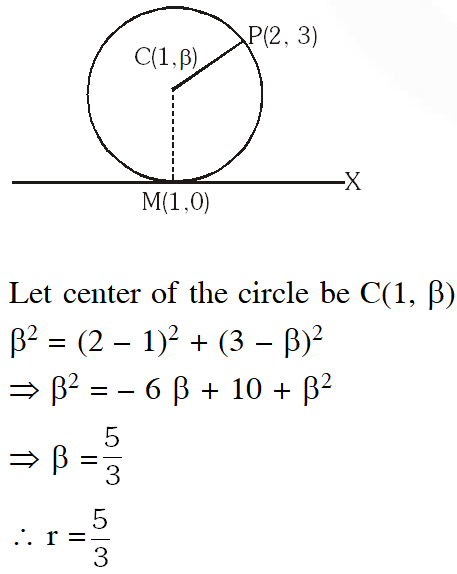diameter $=\frac{10}{3}$
Q. The circle passing through (1, – 2) and touching the axis of x at (3, 0) also passes through the point : (1) (–5, 2)          (2) (2, –5)        (3) (5, –2)          (4) (–2, 5) [JEE (Main)-2013]
Ans. (3) Let equation of circle be $(\mathrm{x}-3)^{2}+(\mathrm{y}+\mathrm{r})^{2}=\mathrm{r}^{2} \because$ it passes through $(1,-2)$ $\Rightarrow \mathrm{r}=2$ $\Rightarrow \quad$ circle is $(\mathrm{x}-3)^{2}+(\mathrm{y}+2)^{2}=4$ $\Rightarrow \quad(5,-2)$ Aliter : $(\mathrm{x}-3)^{2}+\mathrm{y}^{2}+\lambda \mathrm{y}=0 \quad \ldots .(1)$ Putting $(1,-2)$ in ( 1) $\Rightarrow \lambda=4$ Required circle is $\mathrm{x}^{2}+\mathrm{y}^{2}-6 \mathrm{x}+4 \mathrm{y}+9=0$ point $(5,-2)$ satisfies the equation the equation
Q. If a circle $C$ passing through $(4,0)$ touches the circle $x^{2}+y^{2}+4 x-6 y-12=0$ externally at a point $(1,-1),$ then the radius of the circle $C$ is :- (1) $\sqrt{57}$ (2) $2 \sqrt{5}$ (3) 4 (4) 5 [JEE (Main)-2013]
Ans. (4) Equation of family of circles is $(\mathrm{x}-1)^{2}+(\mathrm{y}+1)^{2}+\lambda\left(\mathrm{x}^{2}+\mathrm{y}^{2}+4 \mathrm{x}-6 \mathrm{y}-12\right)=0$ Now it passes of through $(4,0)$ we get $\Rightarrow 9+1+\lambda(16+16-12)=0 \Rightarrow \lambda=-\frac{10}{20}=-\frac{1}{2}$ Required equation of circle is $\mathrm{x}^{2}+\mathrm{y}^{2}-2 \mathrm{x}+2 \mathrm{y}+2-\frac{1}{2}\left(\mathrm{x}^{2}+\mathrm{y}^{2}+4 \mathrm{x}-6 \mathrm{y}-12\right)=0$ $\Rightarrow \mathrm{x}^{2}+\mathrm{y}^{2}-8 \mathrm{x}+10 \mathrm{y}+16=0$ radius $=\sqrt{16+25-16}=5$
Q. If the circle $x^{2}+y^{2}-6 x-8 y+\left(25-a^{2}\right)=0$ touches the axis of $x,$ then a equals :- $(1) \pm 4$ $(2) \pm 3$ (3) 0 $(4) \pm 2$ [JEE-Main (on line)-2013]
Ans. (3,4)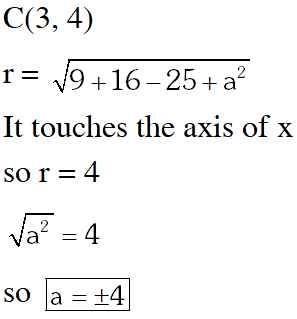Q. Statement I : The only circle having radius $\sqrt{10}$ and a diameter along line $2 \mathrm{x}+\mathrm{y}=5$ is $\mathrm{x}^{2}+\mathrm{y}^{2}-6 \mathrm{x}+2 \mathrm{y}=0$ Statement $\mathbf{I I}: 2 \mathbf{x}+\mathrm{y}=5$ is a normal to the circle $\mathrm{x}^{2}+\mathrm{y}^{2}-6 \mathrm{x}+2 \mathrm{y}=0$. [JEE-Main (on line)-2013] (1) Statement I is false, Statement II is true (2) Statement I is true ; Statement II is false. (3) Statement I is true, Statement II is true, Statement II is not a correct explanation for Statement I. (4) Statement I is true : Statement II is true ; Statement II is a correct explanation for Statement I. [JEE-Main (on line)-2013]
Ans. (1) Normal passes through centre of circle but there can be more then one circle.
Q. Let C be the circle with centre at (1, 1) and radius = 1. If T is the circle centred at (0, y), passing through origin and touching the circle C externally, then the radius of T is equal to : (1) $\frac{\sqrt{3}}{\sqrt{2}}$ ( 2)$\frac{\sqrt{3}}{2}$ (3) $\frac{1}{2}$ (4) $\frac{1}{4}$ [JEE(Main)-2014]
Ans. (4) $\mathrm{C}_{1} \mathrm{C}_{2}=\mathrm{r}_{1}+\mathrm{r}_{2}$ $\sqrt{1+(1-\mathrm{y})^{2}}=1+\mathrm{y}$ $\therefore \mathrm{y}=\frac{1}{4}$ $\therefore \mathrm{radius}=\frac{1}{4}$
Q. The number of common tangents to the circle $x^{2}+y^{2}-4 x-6 y-12=0$ and $x^{2}+y^{2}+6 x$ $+18 y+26=0,$ is : (1) 3             (2) 4               (3) 1               (4) 2 [JEE(Main)-2015]
Ans. (1)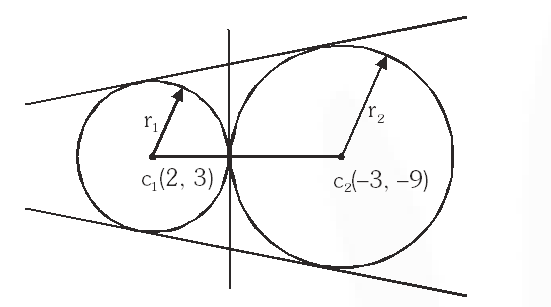distance between centres $\left(\mathrm{c}_{1} \mathrm{c}_{2}\right)=13$ $\mathrm{r}_{1}=\sqrt{4+9+12}=5 \& \mathrm{r}_{2}=\sqrt{9+81-26}=8$ $\mathrm{c}_{1} \mathrm{c}_{2}=\mathrm{r}_{1}+\mathrm{r}_{2}$ is a case of external touching (as shown in above figure), so three common tangentscan be drawn.
Q. If one of the diameters of the circle, given by the euqation, $x^{2}+y^{2}-4 x+6 y-12=0,$ is a chord of a circle $S,$ whose centre is at $(-3,2),$ then the radius of $S$ is : $-$ (1) 10 (2) $5 \sqrt{2}$ (3) $5 \sqrt{3}$ (4) 5 [JEE(Main)-2016]
Ans. (3)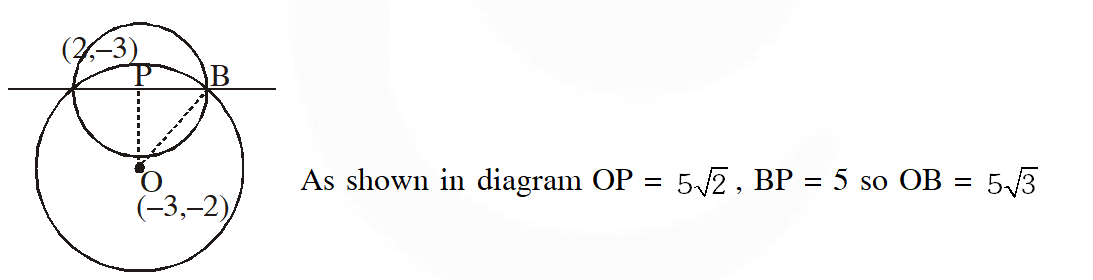Q. The centres of those circles which touch the circle, $x^{2}+y^{2}-8 x-8 y-4=0,$ externally and also touch the $x$ -axis, lie on :- (1) A parabola (2) A circle (3) An ellipse which is not a circle (4) A hyperbola [JEE(Main)-2016]
Ans. (1)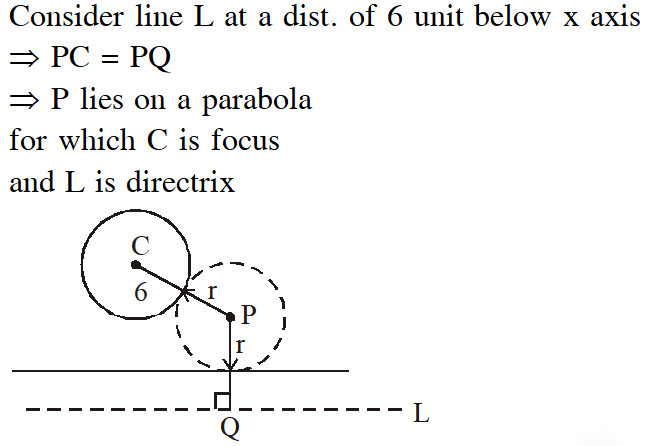Q. If the tangent at $(1,7)$ to the curve $x^{2}=y-6$ touches the circle $x^{2}+y^{2}+16 x+12 y+c=0$ then the value of $c$ is : (1) 185         (2) 85            (3) 95            (4) 195 [JEE(Main)-2018]
Ans. (3)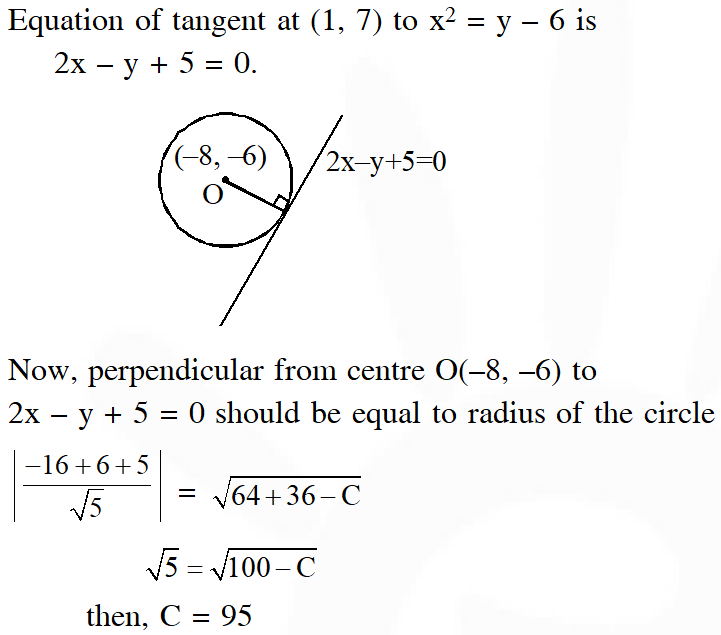Shivam
June 26, 2021, 11:45 a.m.
Nice
aman
May 9, 2021, 7:36 p.m.
wow very nice. Loved it. It is best before giving test
Sai
March 25, 2021, 5:13 p.m.
Very nice 👍 It's like a quize
Mayank garg
March 24, 2021, 11:54 p.m.
ROSHAN
Jan. 5, 2021, 10:38 a.m.
Abhinesh
Dec. 30, 2020, 10:57 a.m.
Carry minati
Sept. 29, 2020, 2:07 p.m.
Neeraja
Sept. 11, 2020, 10:06 a.m.
saivarshithkavali
Sept. 7, 2020, 10:04 p.m.
nice explanation
Bassi Amol
Aug. 28, 2020, 11:43 a.m.
Hii sir very nice esaral pattern
Manoj
Aug. 24, 2020, 9:38 a.m.
Thanks sir
Eswar
Aug. 20, 2020, 3:26 p.m.
Super
Uday Krishna
Aug. 3, 2020, 5:21 p.m.
These questions are good but you need to update to upload recent years also
yamini
July 29, 2020, 8:58 p.m.
thank u for this questions hope this will help in my exams
Vishwanth sai
July 23, 2020, 7:13 p.m.
Questions are lovely
Israel
July 20, 2020, 9:31 a.m.
Update 2019 and 2020 questions
Samiran Dilipkumar Kulkarni
July 19, 2020, 7:54 a.m.
Ramu Kaka
July 12, 2020, 4:40 p.m.
𝑆ℎ𝑟𝑒𝑦𝑎_𝑟𝑎𝑗
July 1, 2020, 11:07 a.m.
𝑈𝑝𝑑𝑎𝑡𝑒 𝐽𝐸𝐸 𝑀𝐴𝐼𝑁𝑆 2020...
𝑆ℎ𝑟𝑒𝑦𝑎_𝑟𝑎𝑗
July 1, 2020, 11:06 a.m.
𝑃𝑙𝑧𝑧 𝑢𝑝𝑑𝑎𝑡𝑒 𝐽𝐸𝐸 𝑀𝐴𝐼𝑁𝑆 2020..
Abdul Mazeed .Akalsabgari
June 30, 2020, 9:20 p.m.
Bindu varsha
June 23, 2020, 7:43 p.m.
🙂
AKASH
June 23, 2020, 12:24 p.m.
June 11, 2020, 6:21 p.m.
Very useful. Thanks 😊